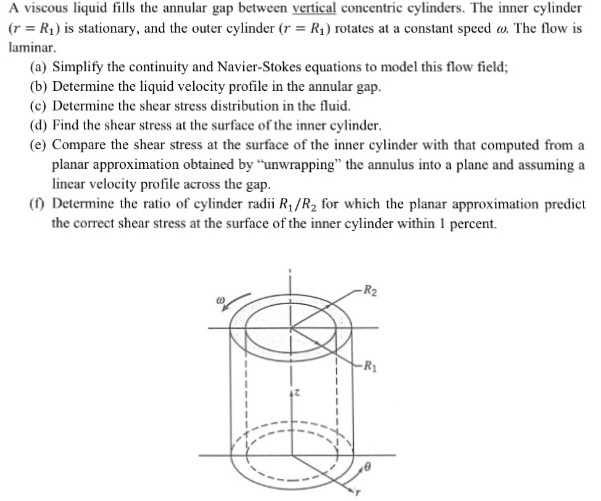### Create an Account

Already have account?

### Forgot Your Password ?

Home / Questions / A viscous liquid fills the annular gap between vertical concentric cylinders The inner cy...

# A viscous liquid fills the annular gap between vertical concentric cylinders The inner cylinder r R1 is stationary and the outer cylinderA viscous liquid fills the annular gap between vertical concentric cylinders. The inner cylinder (r = R_1) is stationary, and the outer cylinder (r = R_1) rotates at a constant speed omega. The flow is laminar. Simplify the continuity and Navier-Stokes equations to model this flow field; Determine the liquid velocity profile in the annular gap. Determine the shear stress distribution in the fluid. Find the shear stress at the surface of the inner cylinder. Compare the shear stress at the surface of the inner cylinder with that computed from a planar approximation obtained by "unwrapping" the annulus into a plane and assuming a linear velocity profile across the gap. Determine the ratio of cylinder radii R_1/R_2 for which the planar approximation predict the correct shear stress at the surface of the inner cylinder within 1 percent.

Apr 29 2020 View more View Less

#### Answer (Solved)Subscribe To Get Solution